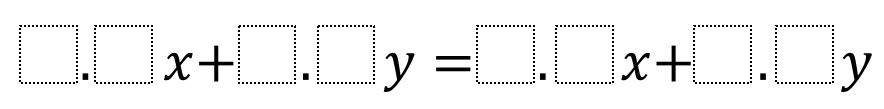# Solving Equations In Two Variables

Directions: Using the digits 1 to 9 at most one time each, fill in the boxes so x = y.### Hint

What needs to happen for us to get to x = y in the last step?

There are many possible solutions including:

9.7x + 4.2y = 8.6x + 5.3y
1.2x + 8.9y = 3.4x + 6.7y
6.8x + 1.3y = 5.7x + 2.4y

Source: Arnav Gulati and Daniel Luevanos

## Difference of Squares and Sum of Cubes

Directions: Using the digits 0 to 9 at most one time each, place a digit …

1.2.3.4.